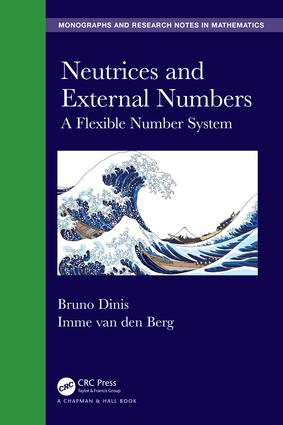# Neutrices and External Numbers

## A Flexible Number System, 1st Edition

Chapman and Hall/CRC

360 pages | 4 B/W Illus.

##### Purchasing Options:\$ = USD
Hardback: 9781498772679
pub: 2019-07-04
SAVE ~\$29.99
Available for pre-order
\$149.95
\$119.96
x

FREE Standard Shipping!

### Description

Neutrices and External Numbers: A Flexible Number System introduces a new model of orders of magnitude and of error analysis, with particular emphasis on behaviour under algebraic operations. The model is formulated in terms of scalar neutrices and external numbers, in the form of an extension of the nonstandard set of real numbers. Many illustrative examples are given. The book starts with detailed presentation of the algebraic structure of external numbers, then deals with the generalized Dedekind completeness property, applications in analysis, domains of validity of approximations of solutions of differential equations, particularly singular perturbations. Finally, it describes the family of algebraic laws characterizing the practice of calculations with external numbers.

Features

• Presents scalar neutrices and external numbers, a mathematical model of order of magnitude within the real number system.
• Outlines complete algebraic rules for the neutrices and external numbers
• Conducts operational analysis of convergence and integration of functions known up to orders of magnitude
• Formalises a calculus of error propagation, covariant with algebraic operations
• Presents mathematical models of phenomena incorporating their necessary imprecisions, in particular related to the Sorites paradox

### Table of Contents

1 Introduction to Elementary Nonstandard Analysis

2 Some models and calculations involving imprecisions.

3 Neutrices and external numbers

4 Advanced properties

5 Sequences. Convergence up to a neutrix

6 Functions of external numbers

7 Integration of functions of external numbers

8 Flexible systems of linear equations

9 Applications in asymptotics

10 Applications in other fields

11 External numbers as a complete arithmetical solid

A Background on Nonstandard Analysis

B Solutions to selected exercises

### About the Authors

Bruno Dinis is a postdoc at the Faculdade de Ciências, University of Lisbon, whose main area of interest is Mathematical Logic, Proof Theory, Nonstandard Analysis, and Philosophy of Mathematics.

Imme van den Berg is Associated Professor in Mathematics at the University of Évora, Portugal. His main area of interest lies in non-standard analysis. He is the author / co-author of 4 books and over 20 articles in the area of non-standard analysis.

### Subject Categories

##### BISAC Subject Codes/Headings:
MAT000000
MATHEMATICS / General
MAT003000
MATHEMATICS / Applied
MAT028000
MATHEMATICS / Set Theory
MAT037000
MATHEMATICS / Functional Analysis